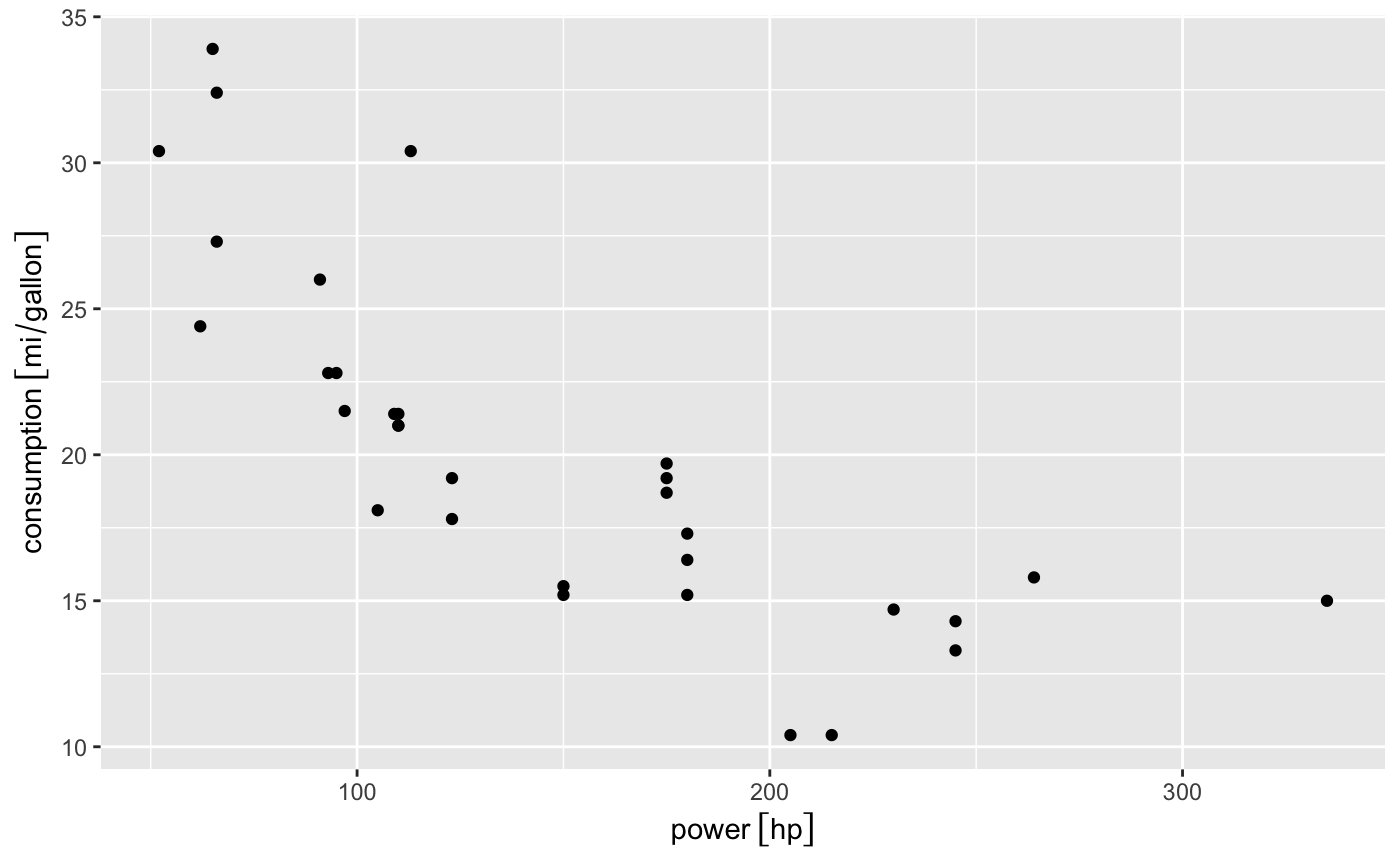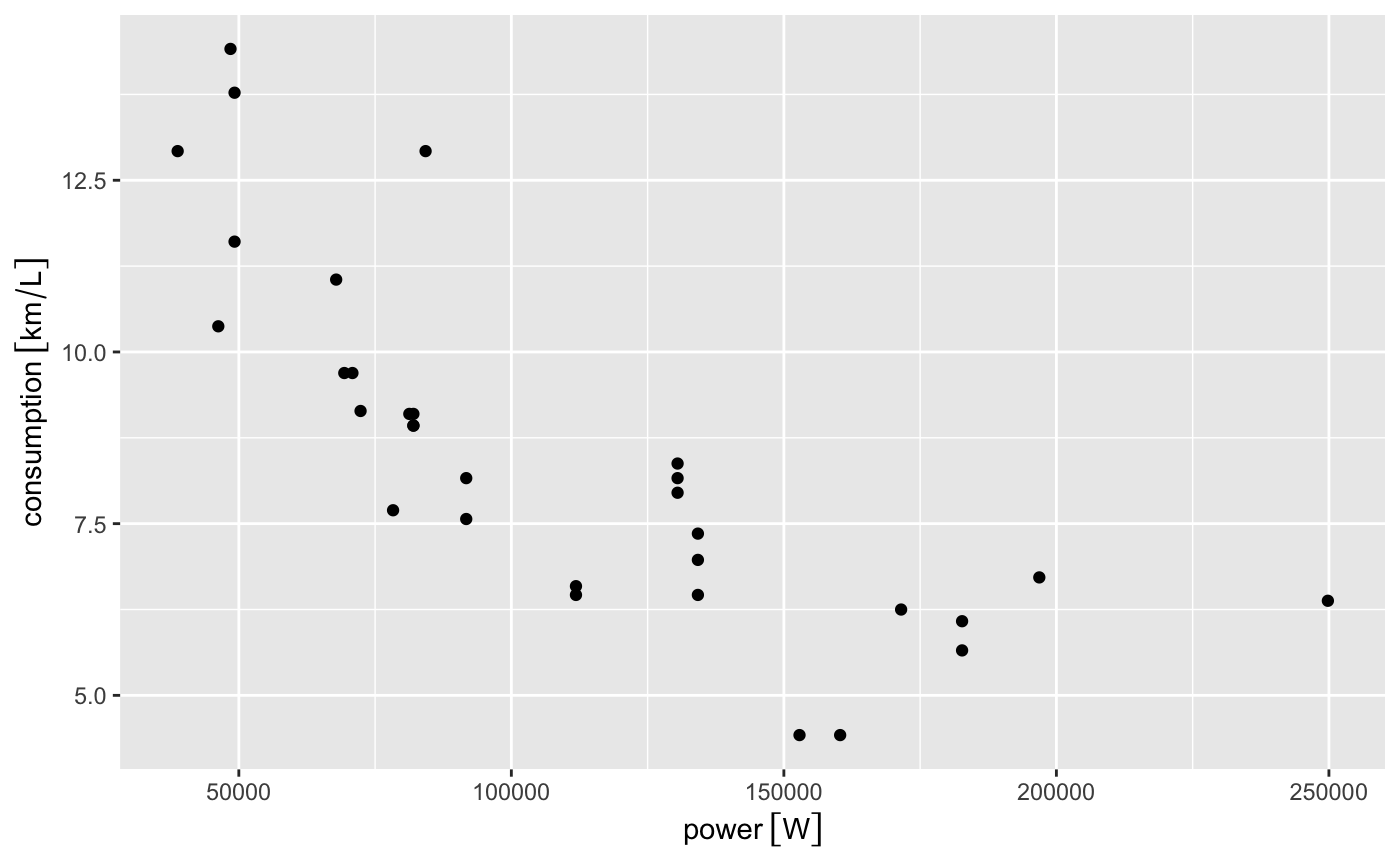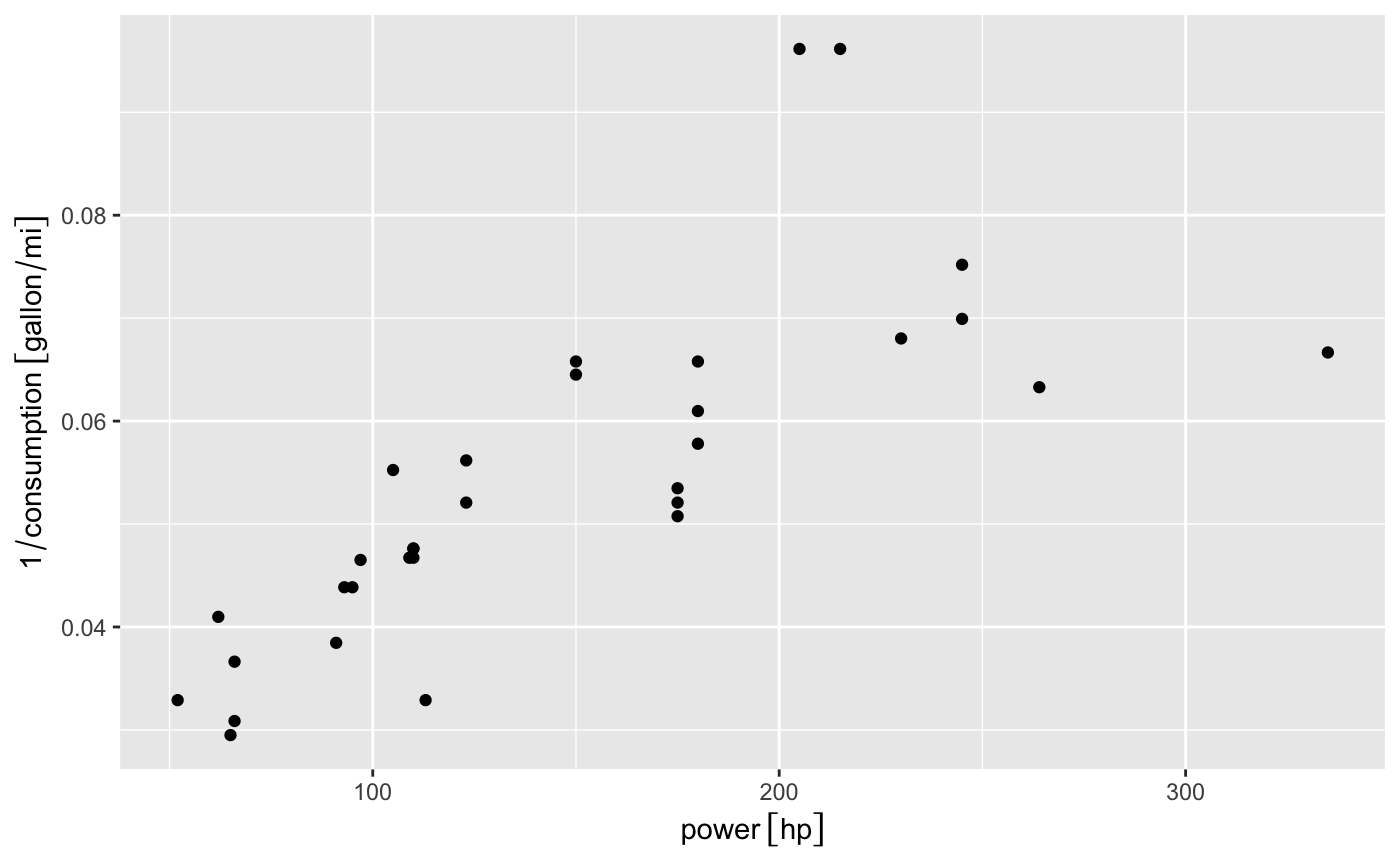These are the default scales for the units class. These will usually be added automatically. To override manually, use scale_*_unit.

scale_x_unit(
name = waiver(),
breaks = waiver(),
unit = NULL,
minor_breaks = waiver(),
labels = waiver(),
limits = NULL,
expand = waiver(),
oob = censor,
na.value = NA_real_,
trans = "identity",
position = "bottom",
sec.axis = waiver()
)

scale_y_unit(
name = waiver(),
breaks = waiver(),
unit = NULL,
minor_breaks = waiver(),
labels = waiver(),
limits = NULL,
expand = waiver(),
oob = censor,
na.value = NA_real_,
trans = "identity",
position = "left",
sec.axis = waiver()
)

## Arguments

name The name of the scale. Used as the axis or legend title. If waiver(), the default, the name of the scale is taken from the first mapping used for that aesthetic. If NULL, the legend title will be omitted. One of: NULL for no breaks waiver() for the default breaks computed by the transformation object A numeric vector of positions A function that takes the limits as input and returns breaks as output (e.g., a function returned by scales::extended_breaks()) A unit specification to use for the axis. If given, the values will be converted to this unit before plotting. An error will be thrown if the specified unit is incompatible with the unit of the data. One of: NULL for no minor breaks waiver() for the default breaks (one minor break between each major break) A numeric vector of positions A function that given the limits returns a vector of minor breaks. One of: NULL for no labels waiver() for the default labels computed by the transformation object A character vector giving labels (must be same length as breaks) A function that takes the breaks as input and returns labels as output One of: NULL to use the default scale range A numeric vector of length two providing limits of the scale. Use NA to refer to the existing minimum or maximum A function that accepts the existing (automatic) limits and returns new limits Note that setting limits on positional scales will remove data outside of the limits. If the purpose is to zoom, use the limit argument in the coordinate system (see coord_cartesian()). For position scales, a vector of range expansion constants used to add some padding around the data to ensure that they are placed some distance away from the axes. Use the convenience function expansion() to generate the values for the expand argument. The defaults are to expand the scale by 5% on each side for continuous variables, and by 0.6 units on each side for discrete variables. One of: Function that handles limits outside of the scale limits (out of bounds). The default (scales::censor()) replaces out of bounds values with NA. scales::squish() for squishing out of bounds values into range. scales::squish_infinite() for squishing infinite values into range. Missing values will be replaced with this value. For continuous scales, the name of a transformation object or the object itself. Built-in transformations include "asn", "atanh", "boxcox", "date", "exp", "hms", "identity", "log", "log10", "log1p", "log2", "logit", "modulus", "probability", "probit", "pseudo_log", "reciprocal", "reverse", "sqrt" and "time". A transformation object bundles together a transform, its inverse, and methods for generating breaks and labels. Transformation objects are defined in the scales package, and are called _trans (e.g., scales::boxcox_trans()). You can create your own transformation with scales::trans_new(). For position scales, The position of the axis. left or right for y axes, top or bottom for x axes. sec_axis() is used to specify a secondary axis.

## Examples

library(units)#> udunits system database from /Users/runner/runners/2.263.0/work/_temp/Library/units/share/udunitsgallon <- as_units('gallon')
mtcars$consumption <- mtcars$mpg * with(ud_units, mi / gallon)
mtcars$power <- mtcars$hp * with(ud_units, hp)

# Use units encoded into the data
ggplot(mtcars) +
geom_point(aes(power, consumption))# Convert units on the fly during plotting
ggplot(mtcars) +
geom_point(aes(power, consumption)) +
scale_x_unit(unit = 'W') +
scale_y_unit(unit = 'km/l')# Resolve units when transforming data
ggplot(mtcars) +
geom_point(aes(power, 1 / consumption))